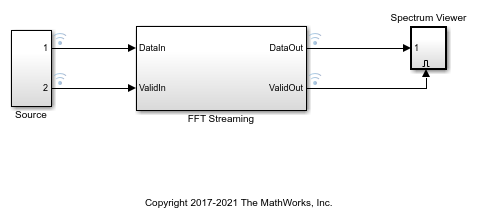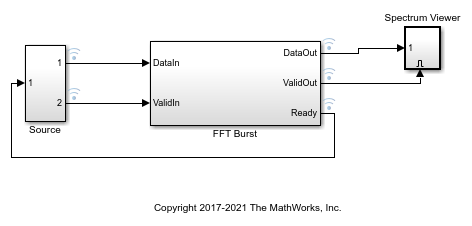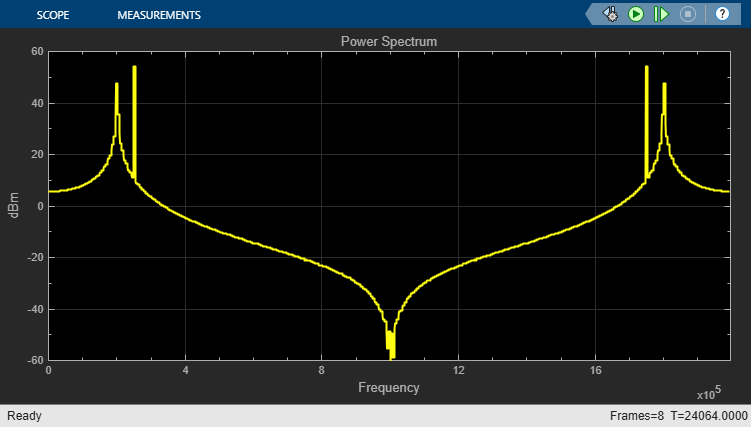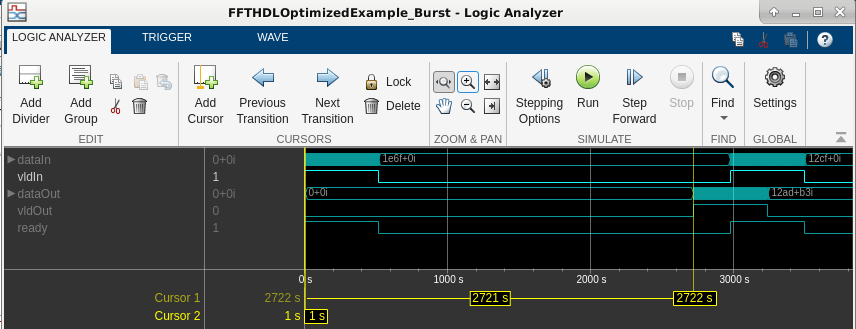# Implement FFT Algorithm for FPGA

This example shows how to implement a hardware-targeted FFT by using DSP HDL Toolbox™ blocks.

Signal processing functions and blocks from DSP System Toolbox™ provide frame-based, floating-point algorithms and can serve as behavioral references for hardware designs. However, efficient hardware designs must use streaming data interfaces and fixed-point data types. Hardware designs also often require control signals such as valid, reset, and backpressure.

The blocks in DSP HDL Toolbox libraries provide hardware-optimized algorithms that model streaming data interfaces, hardware latency, and control signals in Simulink®. The blocks can process a number of samples in parallel to achieve high throughput such as gigasample-per-second (GSPS) rates. You can change the block parameters to explore different hardware implementations. These blocks support HDL code generation and deployment to FPGAs with HDL Coder™.

This example introduces the hardware-friendly streaming data interface and control signals used by DSP HDL Toolbox blocks, and shows how to use the two hardware architectures provided by the FFT block. Then, it shows how to generate HDL code for your design.

The DSP HDL Toolbox FFT block provides two architectures optimized for different use cases. You can set the Architecture parameter on the block to one of these options.

• `Streaming Radix 2^2` — Use this option for high throughput applications. The architecture achieves gigasamples per second (GSPS) when you use vector input.

• `Burst Radix 2` — Use this option for low area applications. The architecture uses only one complex butterfly.

This example includes two models, which show how to use the streaming and burst architectures of the FFT block. The streaming model shows how to use the input and output valid control signals to model data rate independently from the clock rate. The burst model shows how to use the valid control signal to model bursty data streams and how to use a ready signal that indicates when the algorithm can and cannot accept new data samples.

Modern ADCs are capable of sampling signals at sample rates up to several gigasamples per second. However, clock speeds for the fastest FPGA fall short of this sample rate. FPGAs typically run at hundreds of MHz. One way to perform GSPS processing on an FPGA is to process multiple samples at the same time at a much lower clock rate. Many modern FPGAs support the JESD204B standard interface which accepts scalar input at a GHz clock rate, and produces a vector of samples at a lower clock rate. Therefore, modern signal processing requires vector processing.

The `Streaming Radix 2^2` architecture is designed to support high-throughput applications. This example model uses an input vector size of 8 and the Architecture parameter of the FFT block is set to `Streaming Radix 2^2`. For a timing diagram, supported features, and FPGA resource usage, see FFT.

```modelname = 'FFTHDLOptimizedExample_Streaming'; open_system(modelname); ```The InitFcn callback function (Model Properties > Callbacks > InitFcn) sets parameters for the model. In this example, the parameters control the size of the FFT and the input data characteristics.

```FFTLength = 512; ```

The input data is two sine waves, 200 KHz and 250 KHz, each sampled at 1*2e6 Hz. The input vector size is 8 samples.

```FrameSize = 8; Fs = 1*2e6; ```

To demonstrate the use of a valid control signal for noncontinuous input data, this example applies valid input every other cycle.

```ValidPattern = [1,0]; ```

Open the Spectrum Viewer and run the example model.

```open_system('FFTHDLOptimizedExample_Streaming/Spectrum Viewer/Power Spectrum viewer'); set_param(modelname,'SimulationCommand','start') ```Use the Logic Analyzer to view the input and output signals of the `FFT Streaming` subsystem. The waveform shows that the input valid signal is high every second cycle, and that there is some latency before the block returns the first valid output sample. The block mask displays the latency from the first valid input to the first valid output, assuming no gaps in input valid samples. In this case, the actual latency is longer than the displayed latency because of the gaps in the input stream. The block returns the output data with no gaps in the valid samples.### Burst Radix 2 (Minimum Resource) Architecture

Use the `Burst Radix 2` architecture for applications with limited FPGA resources, especially when the FFT length is large. This architecture uses only one complex butterfly to calculate the FFT. In this model, the Architecture parameter of the FFT block is set to `Burst Radix 2`.

When you select this architecture, the block has an output control signal, ready, that indicates when the block can accept new input data. The block sets the ready signal to 1 (`true`) when it can accept data and starts processing once the whole FFT frame is saved into the memory. While processing, the block cannot accept data, so the block sets the ready signal to 0 (`false`). You must apply data only when the ready signal is 1. The block ignores any data applied while the ready signal is 0.

For a timing diagram, supported features, and FPGA resource usage, see FFT.

```modelname = 'FFTHDLOptimizedExample_Burst'; open_system(modelname); ```The InitFcn callback function (Model Properties > Callbacks > InitFcn) sets parameters for the model. In this example, the parameters control the size of the FFT and the input data characteristics.

```FFTLength = 512; ```

The input data is two sine waves, 200 KHz and 250 KHz, each sampled at 1*2e6 Hz. Data is valid every cycle.

```Fs = 1*2e6; ValidPattern = 1; ```

Open the Spectrum Viewer and run the example model.

```open_system('FFTHDLOptimizedExample_Burst/Spectrum Viewer/Power Spectrum viewer'); set_param(modelname,'SimulationCommand','start') ```Use the Logic Analyzer to view the input and output signals of the `FFT Burst` subsystem. The waveform shows that the input data arrives in bursts of valid samples, and that there is some latency before the block returns the valid output samples. The latency in the waveform matches the latency displayed on the block mask. The block also returns an output ready signal that indicates when it has room to start accepting the next burst of input data. To ensure that the next burst of input data is not applied before the block sets the ready signal to 1 (true), the model uses the ready signal as an enable signal for the input data generation.### Generate HDL Code and Test Bench

You must have the HDL Coder product to generate HDL code for this example. Your model must have a subsystem that is targeted for HDL code generation.

Choose one of the models to generate HDL code and test bench for the FFT subsystem.

```systemname = 'FFTHDLOptimizedExample_Burst/FFT Burst'; ```

or

```systemname = 'FFTHDLOptimizedExample_Streaming/FFT Streaming'; ```

Then, use this command to generate HDL code for that subsystem. The generated code can be used for any FPGA or ASIC target.

```makehdl(systemname); ```

Use this command to generate a test bench that compares the results of an HDL simulation against the Simulink simulation behavior.

```makehdltb(systemname); ```